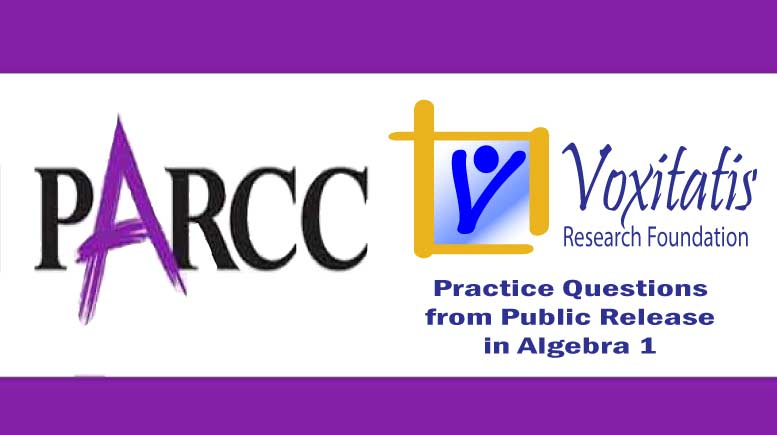Sunday, April 18, 2021# Algebra 1 PARCC question: multiply binomials

-#### The following fill-in-the-blank question, explained here in hopes of helping algebra students in Maryland and Illinois prepare for the PARCC test near the end of this school year, appears on the released version of PARCC’s Spring 2015 test in algebra 1, here:

Multiply the polynomials$(x+3)(2x-4)$

What is the product in the form$ax^2 + bx + c$

a = ___
b = ___
c = ___

Answer and references

Correct answer: a = 2, b = 2, c = –12.

PARCC evidence statement(s) tested: A-APR.1-1: Add, subtract, and multiply polynomials.

The evidence statement above references no Math Practice.

The question tests students’ understanding of the Common Core high school algebra standard HSA.APR.A.1, which states that they should be able to “… add, subtract, and multiply polynomials.” The “APR” acronym in the standards represents “arithmetic with polynomials and rational expressions,” and “HSA” simply means “high school algebra.”

Solution strategy (there are others)

Apply the distributive property by multiplying each term in the first polynomial by each term in the second polynomial and adding the values. Since both polynomials are binomials, we can use the “FOIL” mnemonic device to determine the product:$(x+3)(2x-4)$

For the “F” operation, multiply the first terms of the two binomials: x × 2x = 2x2.

For the “O” operation, multiply the two outer terms of the binomials: x × –4 = –4x.

For the “I” operation, multiply the two inner terms of the binomials: 3 × 2x = 6x.

For the “L” operation, multiply the two last terms: 3 × –4 = –12.

The product of the binomials is the sum of the individual result obtained in this way:$2x^2 - 4x + 6x - 12$$= 2x^2 + 2x - 12$

That expression is in the correct format, and a is simply the coefficient of the x2 term, b is the coefficient of the x term, and c is the constant (including the negative sign).

## Resources for further study

Purple Math, developed by Elizabeth Stapel, a math teacher from the St Louis area, has a few pages about multiplying polynomials. On one of the pages, here, she issues a stern warning about generalizing the FOIL rule: “If you want to use FOIL, that’s fine, but (warning!) keep its restriction in mind: you can ONLY use it for the special case of multiplying two binomials. You can NOT use it at ANY other time!” Good advice.

The Khan Academy, developed by Sal Khan, an engineer who has created a library of thousands of video lessons, has one devoted to the FOIL method, here. In finding the product of two binomials like those in this PARCC practice problem, he says the FOIL method is a way of memorizing something but not really understanding what you’re doing. He’s right. It’s learning a trick to apply the distributive property twice.

For a better method (and more lasting memory of that method, like when you get to age 45), he says, “If we’re multiplying something times an expression, we have to multiply it by every term in that expression.” That’s just logical, and learning it that way will help to drive home the underlying mathematics of what you’re doing when you multiply polynomials.

Chapter 1 of Paul A Foerster’s book Algebra and Trigonometry deals with the multiplication of binomials. He writes, “Once you understand the procedure, you can multiply two binomials quickly, in your head. Just multiply each term of one binomial by each term of the other, and write down the answer.” The book provides a few exercises to practice this skill.

Complete reference: Foerster, Paul A. Algebra and Trigonometry: Functions and Applications, revised edition. Addison-Wesley, 1980, 1984. The book is used in several algebra classes taught in middle and high schools in both Illinois and Maryland.

## Analysis of this question and online accessibility

The question measures knowledge of the Common Core standard it purports to measure and tests students’ ability to multiply two binomials. It is considered to have a low cognitive demand.

The question can be tested online and should yield results that are as valid and reliable as those obtained on paper.

No special accommodation challenges can be identified with this question, so the question is considered fair.

## Challenge

Write a procedure or flowchart for determining the product of three binomials (hint: FOIL won’t work too well in this case). … For determining the product of a binomial and a polynomial with three terms, e.g.,$(2x + 3)(x - 5)(x + 1)$$(x+2)(3x^2-x-5)$

## Purpose of this series of posts

Voxitatis is developing blog posts that address every algebra 1 question released to the public by the Partnership for Assessment of Readiness for College and Careers, or PARCC, in order to help students prepare to take the test this spring.

Our total release will run from February 27 through March 15, with one or two questions discussed per day. Then we’ll move to geometry at the end of March, algebra 2 during the first half of April, and eighth grade during the last half of April.Paul Katulahttps://news.schoolsdo.org
Paul Katula is the executive editor of the Voxitatis Research Foundation, which publishes this blog. For more information, see the About page.

### Md. to administer tests in math, English

0
Students in Md. will still have to take standardized tests this spring in math and English language arts, following action of the state board.